# Rate LawsPage 1

#### WATCH ALL SLIDES

Slide 1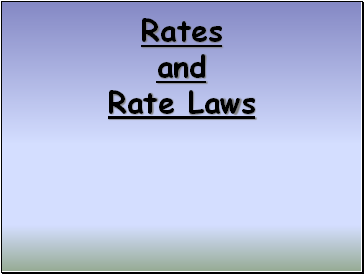Rates and Rate Laws

Slide 2## Reaction Rate

The change in concentration of a reactant or product per unit of time

Slide 32NO2(g)  2NO(g) + O2(g)

Reaction Rates:

2. Can measure

appearance of

products

1. Can measure

disappearance of

reactants

3. Are proportional

stoichiometrically

Slide 4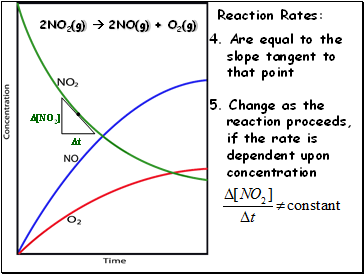2NO2(g)  2NO(g) + O2(g)

Reaction Rates:

4. Are equal to the

slope tangent to

that point

[NO2]

t

5. Change as the

reaction proceeds,

if the rate is

dependent upon

concentration

Slide 5## Rate Laws

Differential rate laws express (reveal) the relationship between the concentration of reactants and the rate of the reaction.

Integrated rate laws express (reveal) the relationship between concentration of reactants and time

The differential rate law is usually just called “the rate law.”

Slide 6Writing a (differential) Rate Law

2 NO(g) + Cl2(g)  2 NOCl(g)

Problem - Write the rate law, determine the value of the rate constant, k, and the overall order for the following reaction:

Slide 7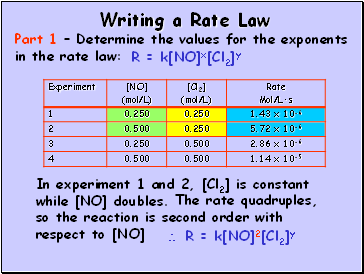Writing a Rate Law

Part 1 – Determine the values for the exponents in the rate law:

In experiment 1 and 2, [Cl2] is constant while [NO] doubles.

R = k[NO]x[Cl2]y

The rate quadruples, so the reaction is second order with respect to [NO]

 R = k[NO]2[Cl2]y

Slide 8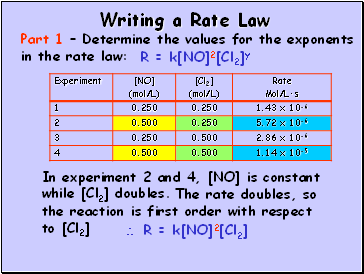Writing a Rate Law

Part 1 – Determine the values for the exponents in the rate law:

R = k[NO]2[Cl2]y

In experiment 2 and 4, [NO] is constant while [Cl2] doubles.

The rate doubles, so the reaction is first order with respect to [Cl2]

 R = k[NO]2[Cl2]

Slide 9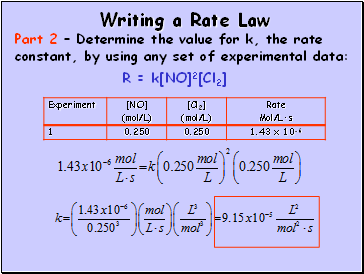Writing a Rate Law

Part 2 – Determine the value for k, the rate constant, by using any set of experimental data:

R = k[NO]2[Cl2]

Slide 10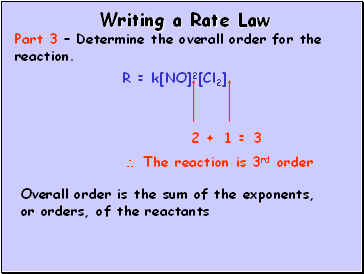Writing a Rate Law

Part 3 – Determine the overall order for the reaction.

R = k[NO]2[Cl2]

Overall order is the sum of the exponents, or orders, of the reactants

Go to page:
1  2